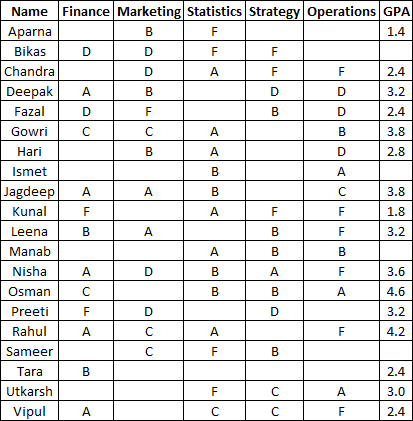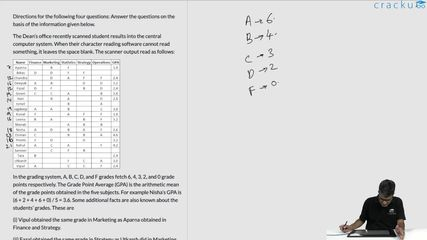### CAT 2004 Question Paper Question 37

Instructions

Directions for the following four questions: Answer the questions on the basis of the information given below.

The Dean’s office recently scanned student results into the central computer system. When their character reading software cannot read something, it leaves the space blank. The scanner output read as follows:In the grading system, A, B, C, D, and F grades fetch 6, 4, 3, 2, and 0 grade points respectively. The Grade Point Average (GPA) is the arithmetic mean of the grade points obtained in the five subjects. For example Nisha’s GPA is (6 + 2 + 4 + 6 + 0) / 5 = 3.6. Some additional facts are also known about the students’ grades. These are

(i) Vipul obtained the same grade in Marketing as Aparna obtained in Finance and Strategy.

(ii) Fazal obtained the same grade in Strategy as Utkarsh did in Marketing.

Question 37

Solution

Since tara received the same grade in exactly 3 subjects , only one possibility is getting B in 3 subjects and F in other 2. So in operation her grade would be either B or F.Among options only manab has B in operations . hence option D.

### View Video Solution